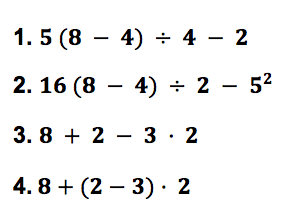Lesson - Order of Operations

Order of Operations

More than one operation may occur in a numerical expression. If the operations were performed in different orders, different answers would result.

For instance, consider 2 + 4 x 5. Different results could occur depending on the order of operations performed.

The Order of Operations is a rule that is applied to ensure that only one answer is possible for a set of operations.

Step 1     Do all operations inside parentheses.

Step 2     Simplify expressions containing exponents.

Step 3     Do multiplication and division as they occur from left to right.

Step 4     Do addition and subtraction as they occur from left to right.

The order is easier to remember if you turn it into an acronym, P E M D A S:

• Parentheses
• Exponents
• Multiplication
• Division
• Subtraction

People have also made up a phrase using the first letter of each word:

"Please Excuse My Dear Aunt Sally"

Practice